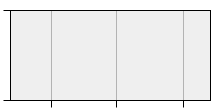# Details

 Table Card RUSMARC
 Title: Reference guide to preparation for entrance control for the discipline "Differential Equations": tutorial Creators: Khakhina Anna M. Organization: Peter the Great St. Petersburg Polytechnic University Imprint: Санкт-Петербург, 2021 Collection: Учебная и учебно-методическая литература; Общая коллекция Subjects: Дифференциальные уравнения; differential equation, formula; function; учебники и пособия для вузов UDC: 517.9(075.8) Document type: Tutorial File type: PDF Language: Russian; English Speciality code (FGOS): 09.03.03 Speciality group (FGOS): 090000 - Информатика и вычислительная техника DOI: 10.18720/SPBPU/5/tr21-218 Rights: Доступ из локальной сети ИБК СПбПУ (чтение, печать) Record key: RU\SPSTU\edoc\67528

Allowed Actions:

Action 'Read' will be available if you login or access site from another network

Group: Anonymous

Network: Internet

## Annotation

The reference manual contains theoretical information and formulas, that are necessary for successfully passing the entrance control in the discipline "Differential Equations", as well as for its further successful study. It is intended for students of all forms of study (full-time, evening and part-time) in the preparation of bachelors in the specialty "Applied informatics” and graduates.

## Document access rights

Network User group Action
ILC SPbPU Local Network AllInternet Anonymous

• LIST OF THOSE YOU NEED TO KNOW FOR SUCCESSFULDEVELOPMENT OF THE COURSE "DIFFERENTIALEQUATIONS "
• A BRIEF REFERENCE ON THE LIST OF THOSE THAT YOU NEED TOKNOW FOR SUCCESSFUL COURSE DEVELOPMENT "DIFFERENTIALEQUATIONS"
• Basic methods for solving equations
• Definitions and properties of degrees and logarithms
• Differentiation of functions of one variable
• Definition of the derivative, geometric meaning
• Derivatives table
• Differentiation rules
• Tangent equation
• Integration
• Integral table
• Various ways of integration
• Matrices and determinants
• Basic definitions
• Calculation of determinants of different orders
• Systems of linear algebraic equations
• Solving System of Equations Using Matrices
• Applications of System of Equations
• Functions of one and several variables
• ADDITIONAL USEFUL INFORMATION FORSUCCESSFUL DEVELOPMENT OF THE COURSE "DIFFERENTIALEQUATIONS "
• Complex numbers
• Algebraic form of a complex number
• Trigonometric form of a complex number
• Solving equations in a set of complex numbers
• Geometric shape of a complex number
• The exponential form of a complex number
• Determinants and matrices. Solving systems of linear equations
• Determinant, properties of determinants, calculation of determinants
• Limits
• Asymptotes of functions
• Differentiation
• Derivatives complex functions. Logarithmic differentiation.

## Usage statisticsAccess count: 1 Last 30 days: 1 Detailed usage statistics